# Real Paper LBO Example

## Introduction

In our prior article, we introduced you to the paper LBO - we covered what a paper LBO is and why it’s part of the private equity interview process. We also provided a comprehensive, step-by-step example.

Below is another practice problem based on a major private equity firm’s modeling test (you might be surprised how infrequently some of these firms update their interview materials). Time yourself - you should be able to complete this exercise in under 5 minutes using a calculator, pen, and paper.

## Assumptions

• You acquire VanillaCo on January 1, 2021 for 1,100. You finance the deal with 500 of equity and 600 of debt. The debt has a 10% interest rate.
• The deal is cash-free, debt-free (no cash or debt on the balance sheet).
• Operating cash flow before interest expense for 2021 is 150, and it increases by 10 each year.
• Ignore the interest expense tax shield (for simplicity).
• Assume no debt paydown; all cash is accumulated on the balance sheet.
• VanillaCo will spend 35 of CapEx each year.
• You sell the company after 5 years for 9.5x LTM unlevered free cash flow (operating cash flow before interest expense - CapEx).

## Questions

1. What is the company’s net debt at exit?
2. Calculate the investor’s returns.
3. Compare the entry multiple and exit multiple.

Try to answer these questions before viewing the solution below.

## Solution

Below is a picture of our pen-and-paper solution, and attached is the Excel version (in case you can’t read our handwriting).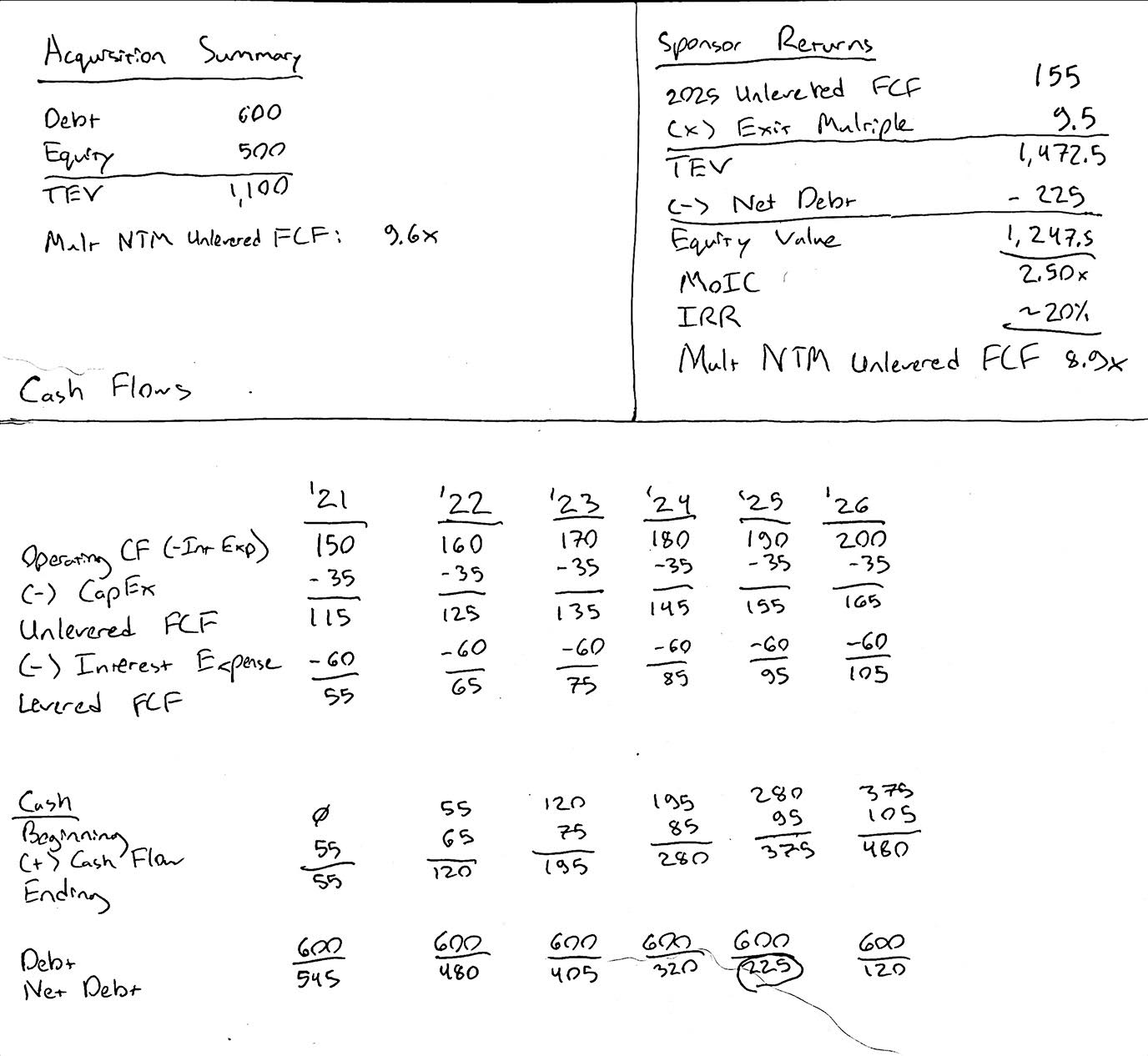1. The company’s net debt at exit (2025) is 225.
2. The company’s equity value at exit is 1,248. The sponsor’s MoIC is 2.50x, and the sponsor’s IRR is 20%.
3. Asking to compare the entry and exit multiples is somewhat of a trick question. Since you don’t know the company’s 2020 financials, you can only calculate a forward entry multiple. That’s why we added an extra year of cash flows in our solution (above). We need the 2026 cash flows in order to derive the forward multiple at exit, so that we can compare the entry and exit multiples on an apples-to-apples basis (+ 5 points for banker jargon). The forward entry multiple is 9.6x (EV / NTM unlevered FCF). The forward exit multiple is 8.9x; therefore, this investment scenario projects multiple contraction. Usually, you would prefer to see multiple expansion, but the fact that the sponsor achieves a 20% IRR anyways is indicative of strong cash flows.

## Step-by-Step Guide

### 1) Set up your template

Below is an image of the template we’re using. You’ll notice we have three basic sections:

• Acquisition Summary
• Sponsor Returns
• Cash Flows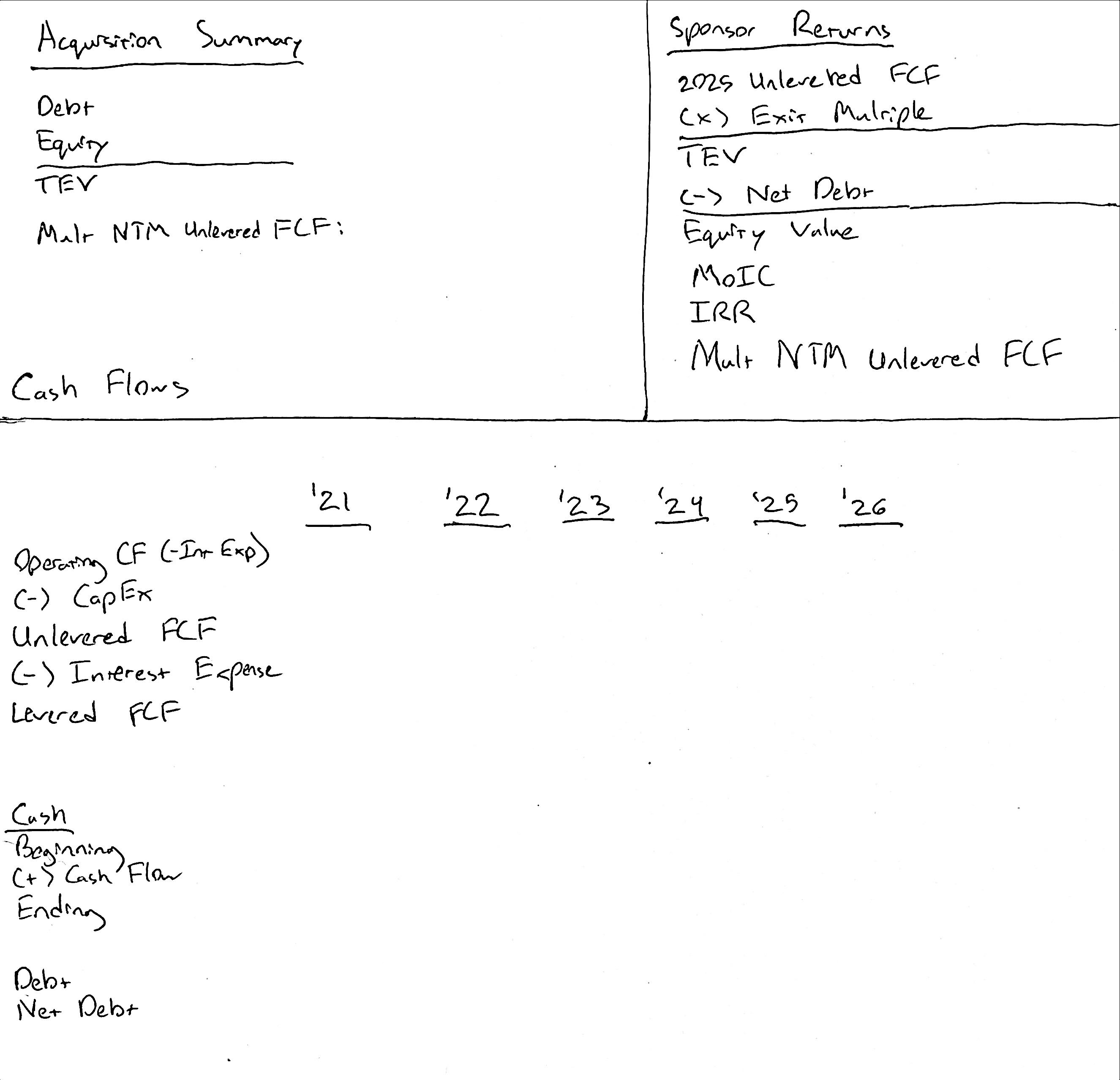Since we’re given the cash flows in the assumptions, we don’t need an income statement.

Also, while it may feel awkward to use free cash flow for calculating multiples, as opposed to EBITDA, remember that’s what EBITDA is supposed to be a proxy for: unlevered FCF.

### 2) Fill out acquisition summary

We can fill out the acquisition summary using the provided assumptions. Below is our updated template: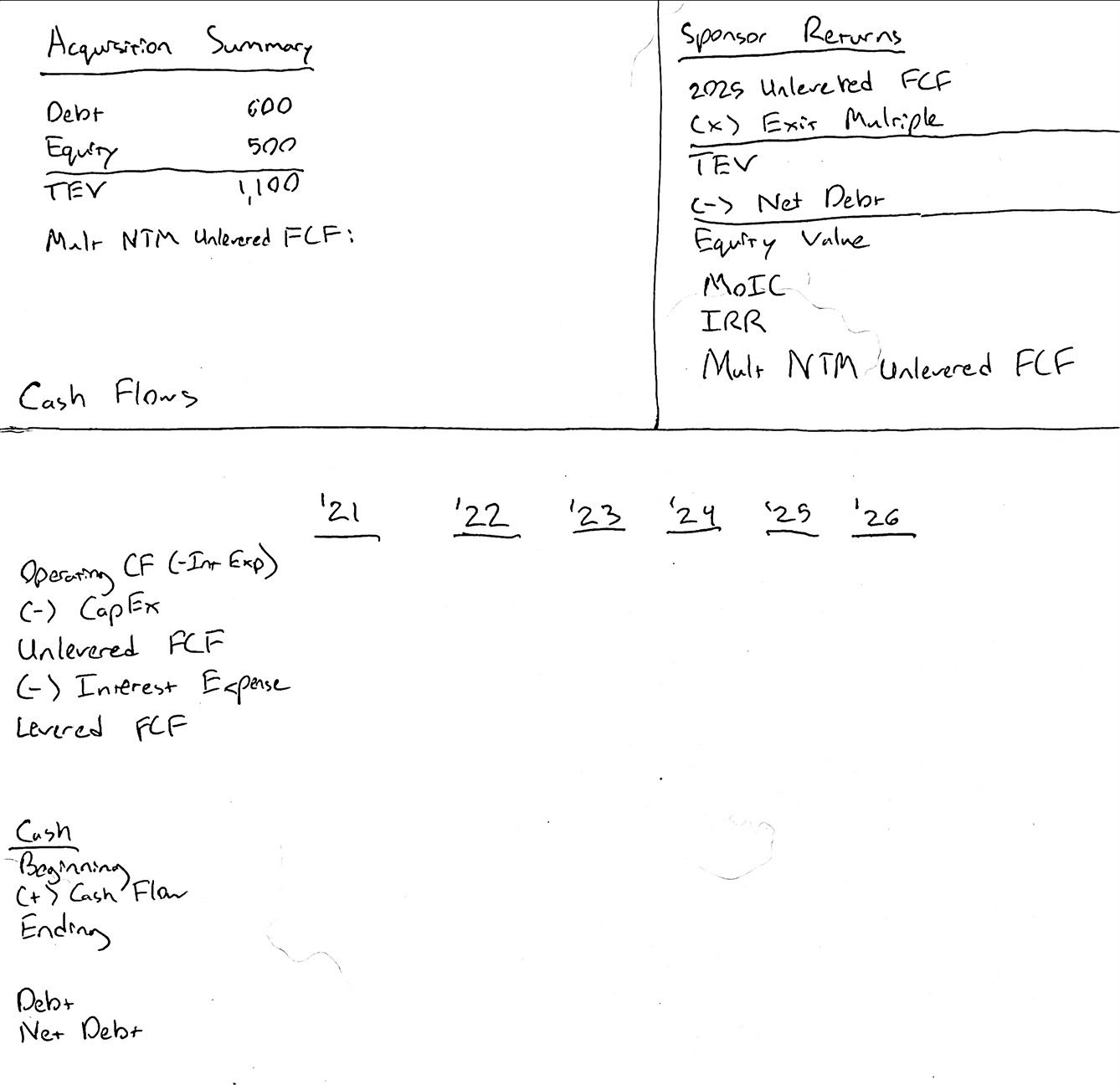We left the NTM entry multiple blank for now.

### 3) Fill out the cash flows

Next we calculate the cash flows. We’re given the 2021 operating cash flow (excluding interest expense), and we’re told the value increases by 10 each year. CapEx is held constant at 35 annually.

Cash Flows Refresher
Levered Free Cash Flow = Cash Flow from Operations - CapEx.

We call it “levered” free cash flow, because cash flow from operations is calculated starting with net income, which includes the impact of interest expense. Therefore, levered free cash flow includes the impact of financial leverage.

Unlevered Free Cash Flow = Levered FCF + Interest Expense - Interest Expense Tax Shield.
Unlevered Free Cash Flow = Cash Flow from Operations (excl. Interest Expense) - CapEx

Rather than paying down debt each year, we’re building a cash balance. Therefore, we can use the same interest expense each year (10% x 600 debt = 60).

We can also add the missing multiple in our acquisition summary (using the 2021 unlevered free cash flow).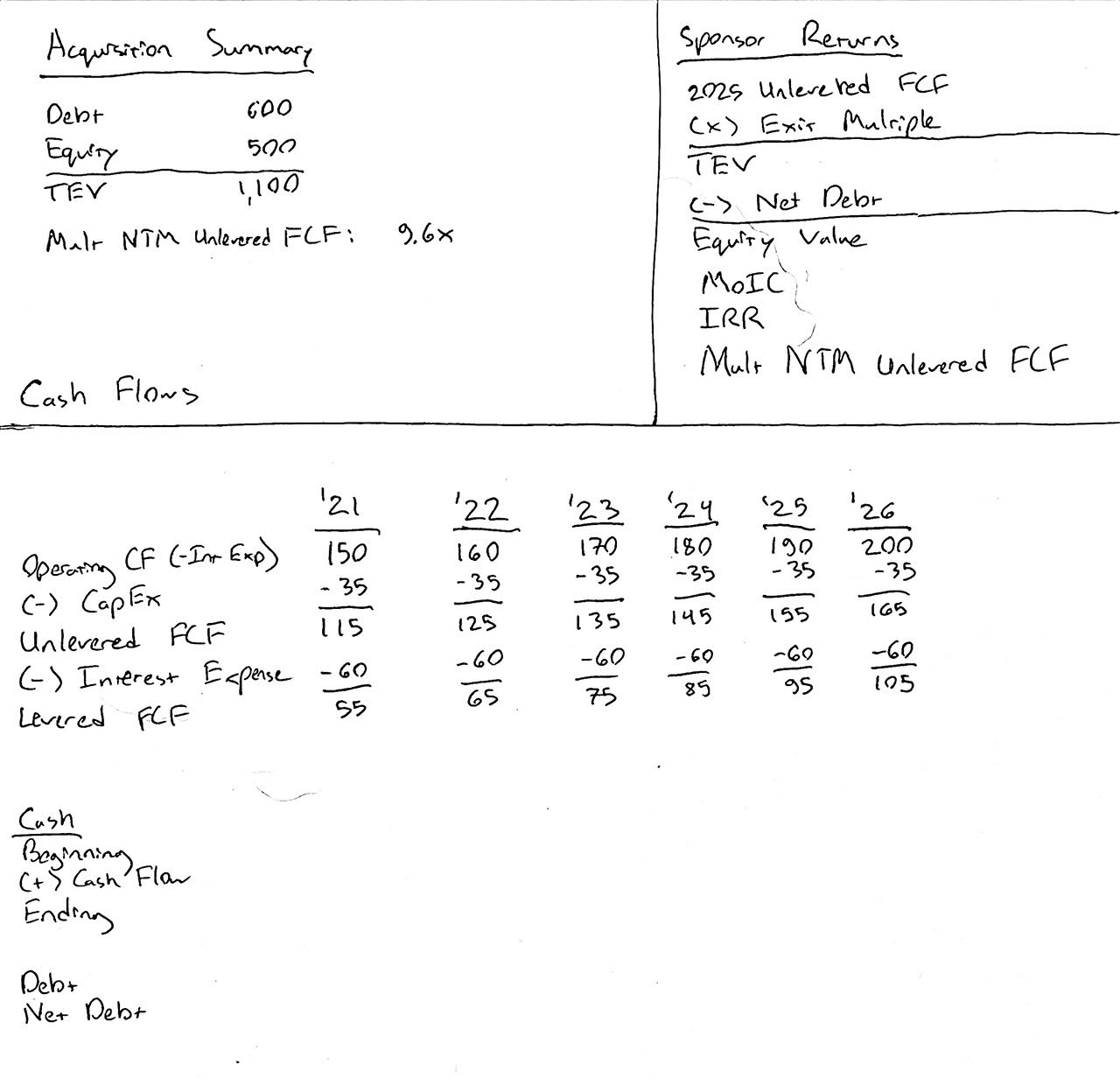### 4) Calculate net debt

This should be straightforward. The debt balance doesn’t change, and cash increases each year.

Below is our updated template: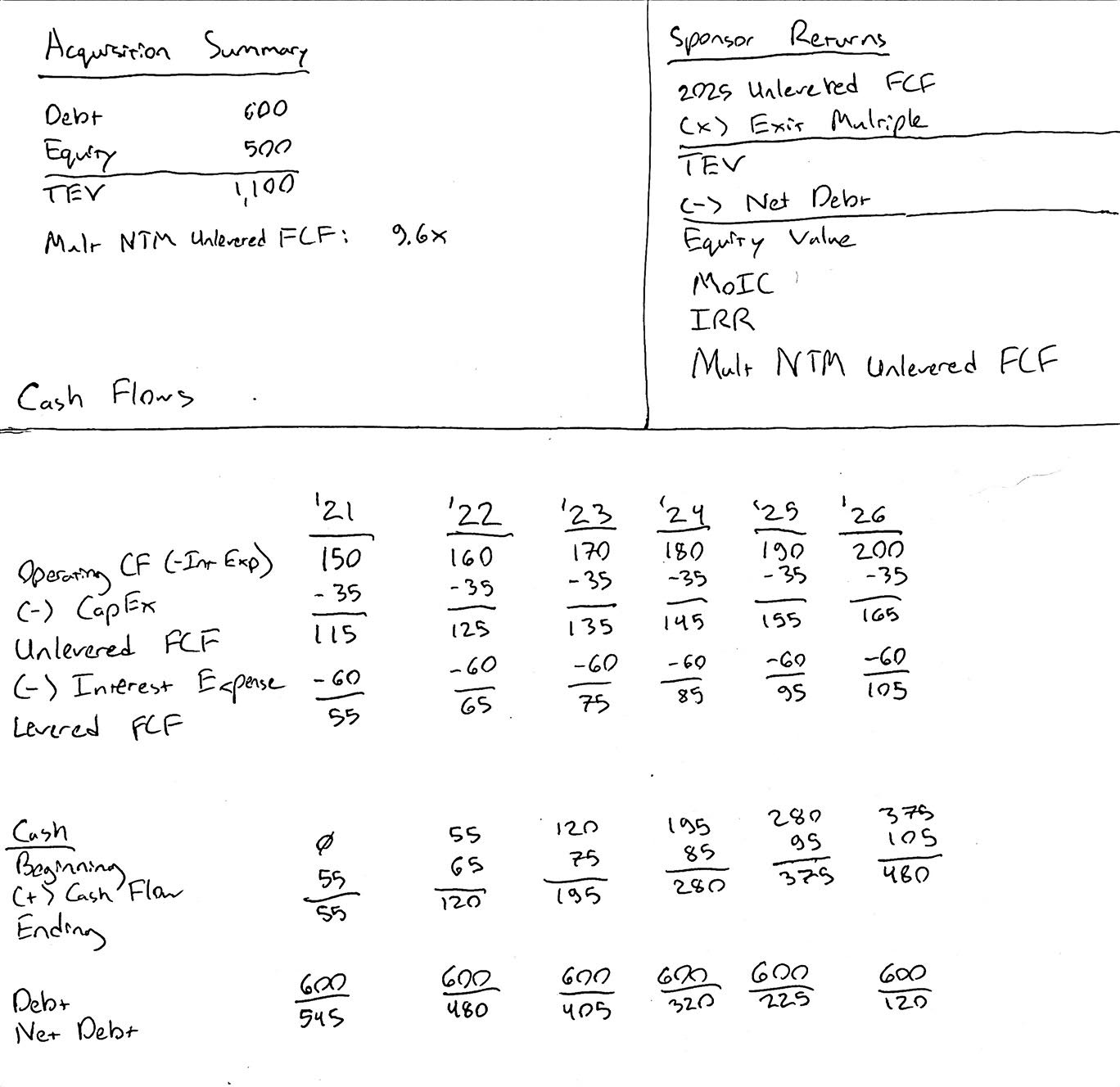### 5) Calculate sponsor returns

The sponsor sells the company after 5 years - at the end of 2025. We calculate the transaction value using an LTM unlevered FCF multiple (9.5x per the assumptions).

Then, we subtract net debt to derive the sponsor equity value.

Sponsor Returns
Whenever you are asked to calculate a sponsor’s returns, you should include both the MoIC and the IRR. If you’re unsure why you should include both, or if you’re a little fuzzy on sponsor returns, check out our intermediate LBO tutorial for a more in-depth discussion.

The MoIC, or Multiple of Invested Capital, compares the cash earned to the cash invested.

MoIC = Cash sponsor receives / Sponsor equity investment

Remember: a shortcut for calculating the IRR uses the MoIC:

IRR = MoIC ^ (1 / years of investment) - 1

But this shortcut only works when there’s a single investment cash flow (i.e., the sponsor didn’t invest more money later on) and a single exit cash flow (i.e., a clean sale with no dividend recaps or earnouts).

Comparing Multiples
One of the questions above asked us to compare the entry and exit multiples. This was a bit of a trick question, because we can only calculate a forward multiple for the initial transaction. Therefore, we need to add an extra year of cash flows (2026), so that we can calculate an implied forward multiple at exit and properly compare the two. Dividing the exit transaction value by our 2026 unlevered free cash flow, we get an implied forward multiple of 8.9x. Therefore, this investment scenario is showing modest multiple contraction, since the forward multiple at exit is less than the initial forward multiple.

Below is our completed template:## Next Steps

Nice job working through this tutorial!

If you’re preparing for private equity interviews, you should practice this problem weekly until you can complete it in less than 5 minutes. Also, make sure you understand the concepts it’s testing:

• Levered vs. unlevered FCF
• Sponsor returns calculations (MoIC, IRR, etc.)
• Comparing multiples on an apples-to-apples basis (forward multiples shouldn’t be compared to LTM multiples)
• Multiple expansion

Check out some of our other tutorials (all free):